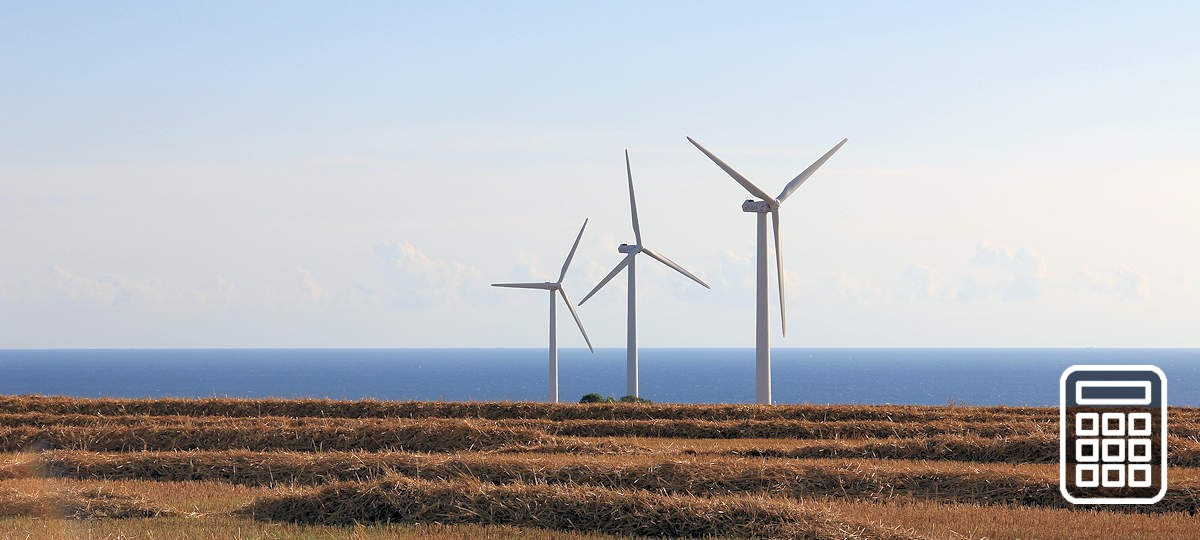### Wind Park Performance Analysis

Name

#### Windpark

Wind power plant
Nominal power PN
kW
Rotor diameter drotor
m
Number of turbines n
m
%

#### Economic Parameters

Investment cost
€/kW
O&M cost
%/a of investment
Plant operating life
years
Interest rate
%Wind Power Plant Characteristics
Grafiktool: Highcharts.com

1 m/s
kW
2 m/s
kW
3 m/s
kW
4 m/s
kW
5 m/s
kW
6 m/s
kW
7 m/s
kW
8 m/s
kW
9 m/s
kW
10 m/s
kW
11 m/s
kW
12 m/s
kW
13 m/s
kW
14 m/s
kW
15 m/s
kW
16 m/s
kW
17 m/s
kW
18 m/s
kW
19 m/s
kW
20 m/s
kW
21 m/s
kW
22 m/s
kW
23 m/s
kW
24 m/s
kW
25 m/s
kW
26 m/s
kW
27 m/s
kW
28 m/s
kW
29 m/s
kW
30 m/s
kW

#### Info: Wind Speed

For the calculations of the wind park performance analysis the mean wind speed in hub height is needed. If wind speed is only given in another measurement height, it can be converted to the hub height using the roughness length.

height h1 above ground
m
roughness length z0
m
wind speed v1 in h1
m/s
hub height h2
m
wind speed. v2 in h2
m/s

Formula used for the calculations:
v2 = v1 · ln (h2/z0) / ln (h1/z0)

#### Info: Air Density

For the calculation of the power coefficient (amount of wind energy used by the converter) the mean air density is needed. The air density is dependent on the ambient temperature and the air pressure. Air pressure and density decrease with the height above sea level. In this form you can estimate the parameters.

Height h above NN
m
Air temperature ϑ
°C
Air pressure p
hPa
Air density ρ
kg/m3

Formulas used for the calculations:
p = 1013 hPa · exp (-h / 8005 m)
ρ = p / 287 J/(kgK) / (273,15 K + ϑ)

#### Info: Park Efficiency

In a wind park the wind converters can block the wind from each other. That reduces the amount of wind energy available to the respective converters. This reduction can be considered by the field efficiency. A real estimation of the field efficiency is complicated and cannot be done here. Nevertheless, you can estimate the efficiency for the calculations.

#### Info: Availability

Faults and maintenance work can result in the failure of some wind converters for certain time periods. Planning errors, a wrong estimated wind speed or other problems also can cause a reduced output. All these effects can be considered by the availability parameter.

#### Explanation of Calculation

distribution:
h(v) = π/2 · v / (vm2) · exp (-π/4·(v/vm)2)

Power coefficient:
cp = 2 · Pel / (ρ · Arotor · v3)

Mean power:
Pm = ηFeld · ηV · Σi ( P(vi) · h(vi) )

Annual generation:
Ea = Pm · 8760 h